Extending operators over generalized nets

Extending operators over generalized nets (not to be confused with the expanding kind of hierarchical operators) are operators that add supplementary functionality to generalized nets.

The operators have at least one of the following two forms:

• local form :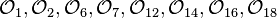$\mathcal{O}_1, \mathcal{O}_2, \mathcal{O}_6, \mathcal{O}_7, \mathcal{O}_{12}, \mathcal{O}_{14}, \mathcal{O}_{16}, \mathcal{O}_{18}$
• global form :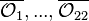$\overline{\mathcal{O}_1}, ..., \overline{\mathcal{O}_{22}}$

The extending operators are:

• operators, extending a given generalized net (GN) to a fuzzy and intuitionistic fuzzy generalized net: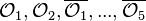$\mathcal{O}_1, \mathcal{O}_2, \overline{\mathcal{O}_1},..., \overline{\mathcal{O}_5}$,
• an operator, replacing the firing moment of a given transition in a given GN with an interval :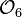$\mathcal{O}_6$,
• and a global operator, replacing the firing moments of all transitions in the GN with intervals :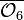$\overline{\mathcal{O}_6}$,
• and operators doing the opposite :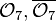$\mathcal{O}_7, \overline{\mathcal{O}_7}$.
• an operator colouring the tokens and the arcs of a given GN :$\overline{\mathcal{O}_8}$,
• and an operator, doing the opposite :$\overline{\mathcal{O}_9}$.
• an operator which adds a "memory" component to the structure of a given GN :$\overline{\mathcal{O}_{10}}$,
• and one removing this component :$\overline{\mathcal{O}_{11}}$.
• operators transforming a given GN to a GN with optimization components :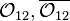$\mathcal{O}_{12}, \overline{\mathcal{O}_{12}}$,
• and an operator that removes such components from the net :$\overline{\mathcal{O}_{13}}$.
• operators transforming a given GN to a GN with additional clocks :$\mathcal{O}_{14}, \overline{\mathcal{O}_{14}}$,
• and an operator that removes the existing additional clocks from a GN :$\overline{\mathcal{O}_{15}}$.
• operators transforming a given GN to a GN with complex transition types :$\mathcal{O}_{16}, \overline{\mathcal{O}_{16}}$,
• and an operator that removes these components :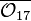$\overline{\mathcal{O}_{17}}$.
• operators transforming a given GN to a GN with transition stop conditions :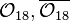$\mathcal{O}_{18}, \overline{\mathcal{O}_{18}}$,
• and one that removes these conditions from the net :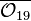$\overline{\mathcal{O}_{19}}$.
• an operator transforming a given GN to a GN with (global) stop condition :$\overline{\mathcal{O}_{20}}$,
• and one that removes this conditions from the net :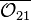$\overline{\mathcal{O}_{21}}$.
• an operator that transforms a given GN to a two-way generalized net :$\overline{\mathcal{O}_{22}}$.